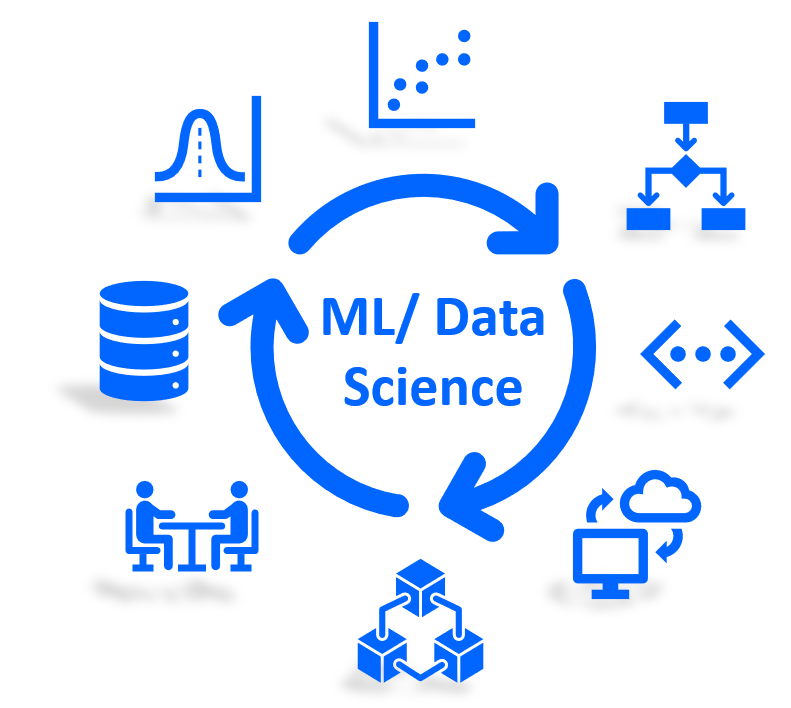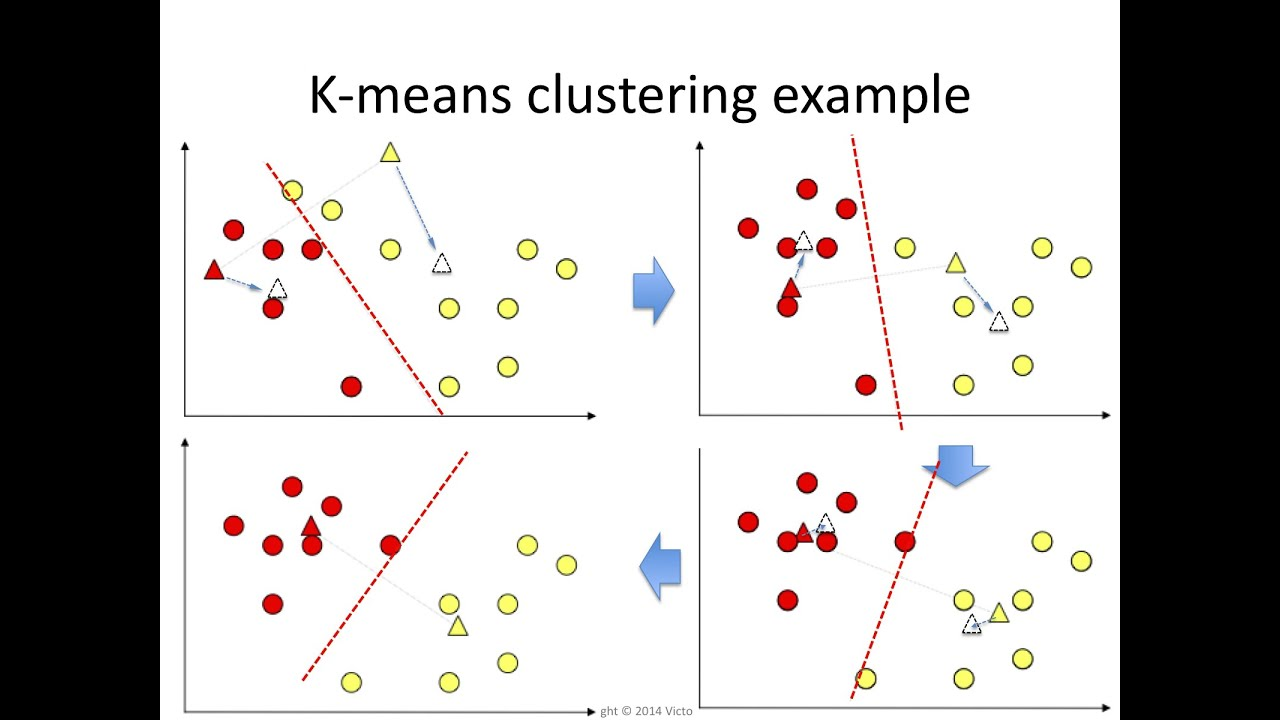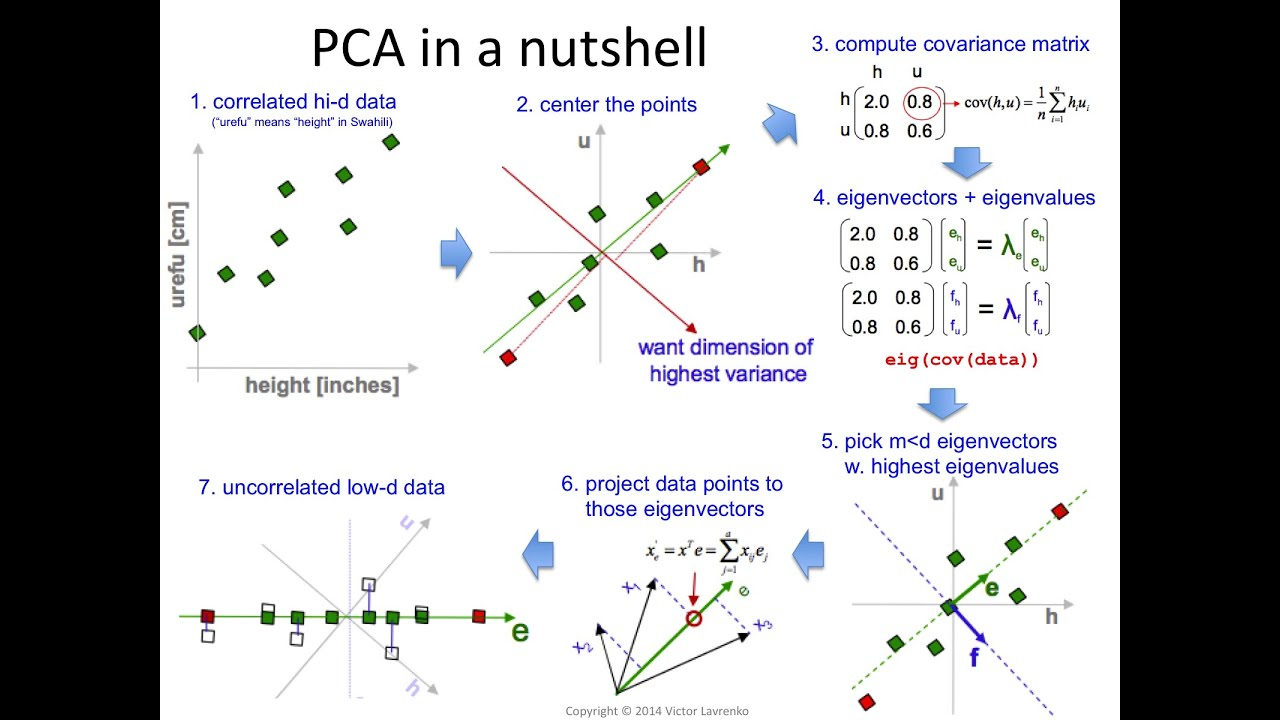Python Machine Learning Jupyter Notebooks (ML website)

Dr. Tirthajyoti Sarkar, Fremont, California (Please feel free to connect on LinkedIn here)Requirements

• Python 3.6+
• NumPy (pip install numpy)
• Pandas (pip install pandas)
• Scikit-learn (pip install scikit-learn)
• SciPy (pip install scipy)
• Statsmodels (pip install statsmodels)
• MatplotLib (pip install matplotlib)
• Seaborn (pip install seaborn)
• Sympy (pip install sympy)
• WTForms (pip install wtforms)
• Tensorflow (pip install tensorflow>=1.15)
• Keras (pip install keras)
• pdpipe (pip install pdpipe)

"Some Essential Hacks and Tricks for Machine Learning with Python"Essential tutorial-type notebooks on Pandas and Numpy

Jupyter notebooks covering a wide range of functions and operations on the topics of NumPy, Pandans, Seaborn, Matplotlib etc.

Tutorial-type notebooks covering regression, classification, clustering, dimensionality reduction, and some basic neural network algorithms

Regression

• Simple linear regression with t-statistic generationClassificationClustering• K-means clustering (Here is the Notebook)

• Affinity propagation (showing its time complexity and the effect of damping factor) (Here is the Notebook)

• Mean-shift technique (showing its time complexity and the effect of noise on cluster discovery) (Here is the Notebook)

• DBSCAN (showing how it can generically detect areas of high density irrespective of cluster shapes, which the k-means fails to do) (Here is the Notebook)

• Hierarchical clustering with Dendograms showing how to choose optimal number of clusters (Here is the Notebook)Dimensionality reduction

• Principal component analysisObject-oriented programming with machine learning

Implementing some of the core OOP principles in a machine learning context by building your own Scikit-learn-like estimator, and making it better.

See my articles on Medium on this topic.

Unit testing ML code with Pytest

Check the files and detailed instructions in the Pytest directory to understand how one should write unit testing code/module for machine learning models

Memory and timing profiling

Profiling data science code and ML models for memory footprint and computing time is a critical but often overlooed area. Here are a couple of Notebooks showing the ideas,

Get A Weekly Email With Trending Projects For These Topics
No Spam. Unsubscribe easily at any time.
Jupyter Notebook (241,997
Machine Learning (31,768
Deep Learning (23,738
Data Science (9,206
Neural Network (8,624
Artificial Intelligence (5,569
Statistics (4,338
Pandas (3,941
Numpy (3,499
Classification (3,158
Matplotlib (2,247
Scikit Learn (1,992
Clustering (1,852
Regression (1,346
Random Forest (1,216
Decision Trees (852
Pytest (745
Dimensionality Reduction (362
Naive Bayes (251
K Nearest Neighbours (114
Related Projects